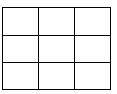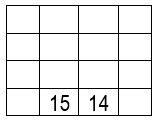# The wonders of the 3 by 3 square (Part 1)

Over a thousand years ago, even before we adopted the Hindu-Arabic numeral system we now use, apparently the Chinese started to explore the problem of placing the integers 1 to 9 into a 3 by 3 grid, in such a way that in each of the 8 ways you could have three squares successively, 3 horizontal, 3 vertical, and 2 diagonal, the totals would be the same. They called this the Lo-Shu square. In Western mathematics it got called a Magic square. Fig 1 is an empty one.

#### Can you find a solution?Fig 1

The process whereby someone new to this task can be helped to find it is a useful one for young learners to go through. It does not help if teacher just gives them a solution! This is an important issue in all 21st century mathematics teaching.
If we write down the numbers 1-9 in order, and find out their total [after all, if each of (say) the three rows totals the same, then each must be on third of the total of the 9 integers].
As we add the numbers, most often comes a pattern the learners recognise: 1, 3, 6, 10, 15… This pattern can reinforce, or prove an interesting diversion to talk about, these Triangular numbers, including why they are so called, and perhaps then how they relate to the Square numbers.
Although at first it may seem that many alternative solutions exist to the order 3 Magic Square, actually, all solutions, allowing for reflections and rotations, still make use of the unique solution.
Many extensions/variations are possible. The most common is to challenge the learners to use the integers 1-16 to make a 4 by 4, or “order 4” Magic Square. Quite a number of different ones of this size exist, but a good first step starting from scratch might be to calculate the Magic Constant, the total of each row, column and diagonal. Can you calculate how to get the value 34? (without using a formula the children don’t know!)
In 1514 Albrecht Dürer (1471-1528) Germany’s elite Renaissance artist incorporated a magic square into his painting entitled “Melancholia”, in which he placed 1514 into 2 of the squares, see Fig 2.Fig 2

Can you complete the 4 by 4 (or order 4) magic square? It’s not easy, even if (Hint!) this square has some delightful properties, many ways in which the total of 4 squares is 34 as well as for the rows, columns and diagonals: for example, the 4 corner squares, or the 4 central squares, add up to 34 too.
Many other order 4 magic squares are possible which are distinct from each other.
There is a strategy for creating odd order Magic Squares which you may like to explore.
A more challenging problem is to find a magic square which is such that from 1 to 16 every move is a chess knight’s move, 2 steps in one direction then one at right angles.

By Bob Vertes - a semi-retired Mathematics teacher and teacher educator.